Young’s Double Slit Experiment

• Last Updated : 20 Oct, 2021

Optics is the part of material science that concentrates on the conduct and properties of light, incorporating its connections with issues and the development of instruments that utilise or recognize it. Optics as a rule depicts the conduct of apparent, bright, and infrared light. Since light is an electromagnetic wave, different types of electromagnetic radiation, for example, X-beams, microwaves, and radio waves show comparative properties. Most optical marvels can be represented by utilizing the traditional electromagnetic portrayal of light.

Complete electromagnetic depictions of light are, in any case, frequently hard to apply practically speaking. Down to earth optics is typically done utilizing worked on models. The most widely recognized of these, mathematical optics, regards light as an assortment of beams that move in straight lines and curve when they go through or reflect from surfaces. Actual optics is a more thorough model of light, which incorporates wave impacts, for example, diffraction and obstruction that can’t be represented in mathematical optics. By and large, the beam based model of light was grown first, trailed by the wave model of light. Progress in the electromagnetic hypothesis in the nineteenth century prompted the disclosure that light waves were indeed electromagnetic radiation.

Young’s Double Slit Experiment

Young’s double-slit experiment employs two coherent light sources separated by a modest distance, generally a few orders of magnitude larger than the wavelength of light.

Young’s double-slit experiment aided in the understanding of lightwave theory. The slits are separated by a large distance ‘D’ from a screen or photodetector. Young’s original double-slit experiment employed diffracted light from a single source that was then transmitted through two additional slits to serve as coherent sources. In today’s investigations, lasers are frequently employed as coherent sources.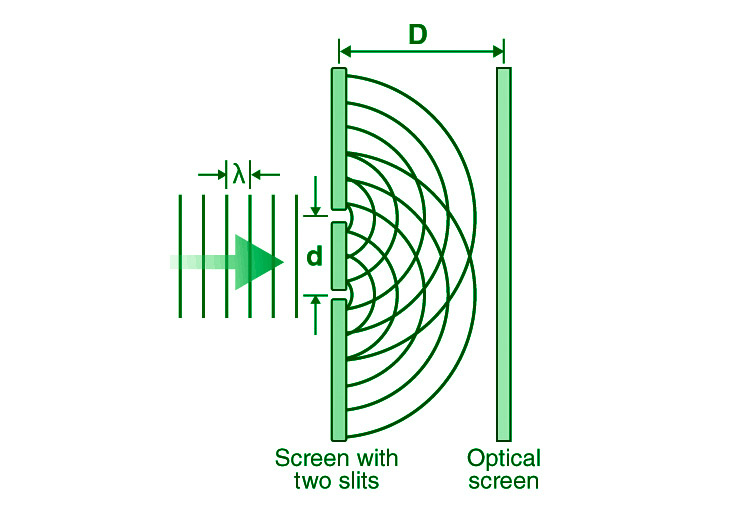Each source may be thought of as a coherent lightwave source. The waves travel lengths l1 and l2 to generate a path difference of l at any place on the screen at a distance ‘y’ from the centre. At the sources, the point roughly subtends an angle of (since the distance D is large there is only a very small difference of the angles subtended at sources).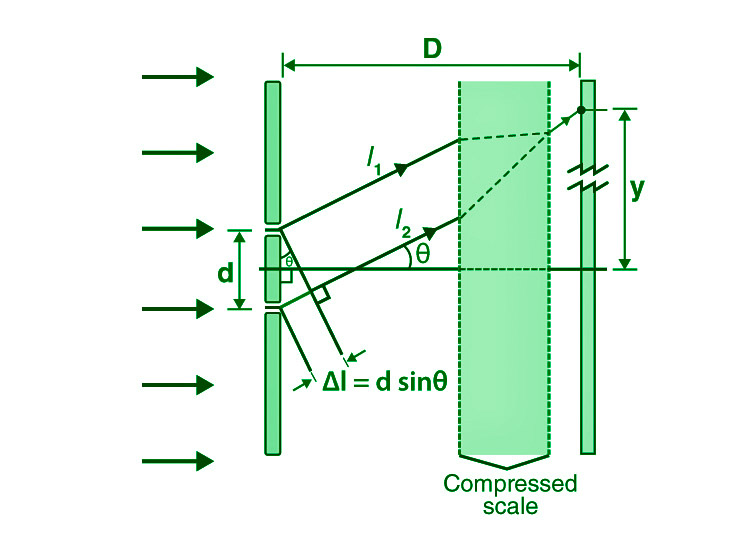Derivation for Young’s Double Slit Experiment

Consider a monochromatic light source ‘S’ that is kept a long way from two slits s1 and s2. S is in the same plane as s1 and s2. Because both s1 and s2 are drawn from S, they act as two consistent sources.

The light travels through these slits and lands on a screen that is positioned at a distance ‘D’ from the apertures s1 and s2. The distance between two slits is denoted by the letter ‘d.’

If s1 is open and s2 is closed, the screen opposite s1 is darkened, leaving just the screen opposite s2 lit. Only when both slits s1 and s2 are open can interference patterns form.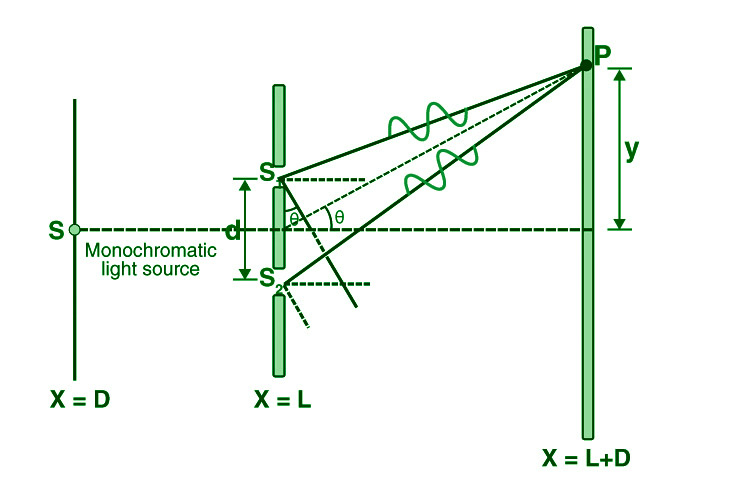At the point when the cut partition (d) and the screen distance (D) are kept unaltered, to arrive at P the light waves from s1 and s2 should travel various distances. It suggests that there is a way contrast in Young’s twofold cut test between the two light waves from s1 and s2.

Approximations in Young’s twofold cut analysis-

Guess 1: D > d

Since D > d, the two light beams are thought to be equal.

Guess 2: d/λ >> 1

Often, d is a small amount of a millimeter and λ is a negligible portion of a micrometer for noticeable light.

Under these conditions θ is little, consequently, we can utilize the estimate,

sin θ = tan θ ≈ θ = λ/d

∴ the path difference,

Δz = λ/d

This is the path difference between two waves meeting at a point on the screen. Because of this path difference in Young’s twofold cut investigation, a few focuses on the screen are brilliant and a few focuses are dull.

Position of Fringes In Young’s Double Slit Experiment

• Position of Bright Fringes

For maximum intensity or bright fringe to be formed at P

Path difference, Δz = nλ (n = 0, ±1, ±2, . . . .)

i.e.

xd/D = nλ

or

x = nλD/d

The distance of the nth  bright fringe from the centre is

xn = nλD/d

Similarly, the distance of the (n-1)th bright fringe from the centre is

x(n-1) = (n -1)λD/d

Fringe width,  β = x – x (n-1) = nλD/d – (n -1)λD/d = λD/d

(n = 0, ±1, ±2, . . . .)

• Position of Dark Fringes

For minimum intensity or dark fringe to be formed at P,

Path difference, Δz = (2n + 1) (λ/2) (n = 0, ±1, ±2, . . . .)

i.e.

x = (2n +1)λD/2d

The distance of the nth  dark fringe from the centre is

xn = (2n+1)λD/2d

Similarly, the distance of the (n-1)th bright fringe from the centre is

x(n-1)= (2(n-1) +1)λD/2d

Fringe width, β = x n  – x (n-1) = (2n + 1) λD/2d – (2(n -1) + 1)λD/2d = λD/d

(n = 0, ±1, ±2, . . . .)

Fringe Width

The fringe width is the distance between two consecutive bright (or dark) fringes.

β = λD/d

When Young’s double-slit experiment equipment is submerged in a liquid with a refractive index of (μ), the wavelength of light and the fringe width both fall by ” times.

β 1= β/μ

When white light is utilised instead of monochromatic light, coloured fringes appear on the screen, with red fringes being bigger than violet fringes.

Maximum Order of Interference Fringes

On the screen, the position of nth order maximum is,

γ = nλD/d

where n=0, ±1, ±2, …

However, because the 2nd approximation would be violated, ‘n’ values cannot take infinitely high values. i.e. θ is small (or) y << D.

⇒ γ/D = nλ/d <<1

As a result, the above formula for interference maxima can be used n<< d/λ. When the value of ‘n’ equals that of d/λ, the path difference can no longer be calculated as dγ/D.

Hence for maxima, path difference =

⇒ dsinθ = nλ

or

​n = dsinθ/λ

and

nmax= d/λ

The above represents the box function or greatest integer function. Similarly, the highest order of interference minima is given by,

​nmin=[d/λ+1/2]

Shape of Interference Fringes in YDSE

The route difference between the two slits is represented by the YDSE diagram.

s2p−s1p≈dsinθ (constant)

The preceding equation depicts a hyperbola with two foci denoted by the letters s1 and s2.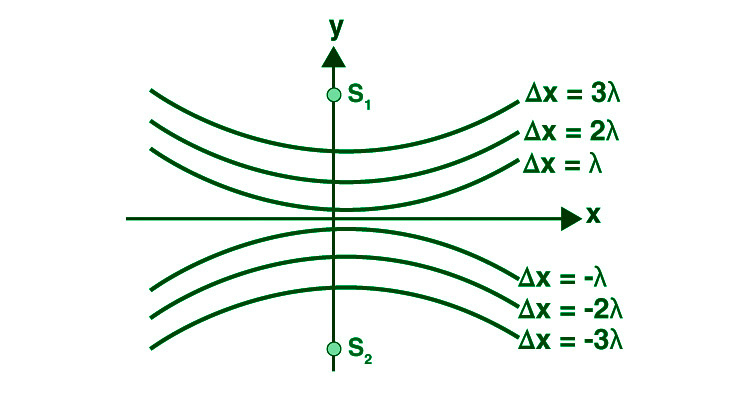When we rotate a hyperbola on the axis s1s2, we obtain an interference pattern on the screen that is a segment of a hyperbola. Fringes are hyperbolic with a straight middle portion of the screen is in the yz plane.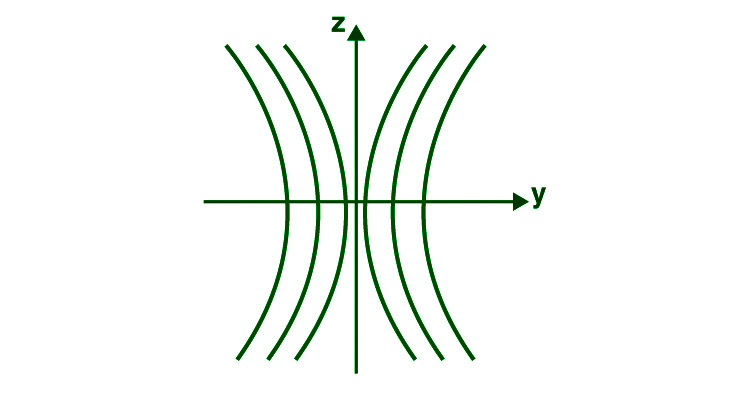Intensity of Fringes in YDSE

The resulting intensity at location p for two coherent sources s1 and s2 is given by

I = I1 + I2 + 2 √(I1 . I2) cos φ

Putting, I1 = I2 = I0 (Since, d<<<D).

I = I0 + I0 + 2 √(I0.I0) cos φ

= 2I0 + 2 (I0) cos φ

= 2I0 (1 +  cos φ)

= 4I0cos2(ϕ/2)

Constructive and Destructive Interference

The path difference must be an integral multiple of the wavelength for constructive interference to occur. As a result, if a brilliant fringe is at ‘y,’

nλ = y d/D

where n = ±0,1,2,3…..

The centre brilliant fringe is represented by the 0th fringe. Similarly, in Young’s double-slit experiment, the expression for a black fringe may be obtained by setting the path difference to:

Δl = (2n+1)λ/2

This simplifies to,

(2n+1)λ/2 = y d/D

or

y = (2n+1)λD/2d

Young’s double-slit experiment was a breakthrough point in science because it proved beyond a shadow of a doubt that light behaved like a wave. Later, the Double Slit Experiment was repeated using electrons, and to everyone’s amazement, the pattern produced was nearly identical to that seen with light. This would forever alter our perceptions of matter and particles, requiring us to believe that matter, like light, acts like a wave.

Sample Questions

Question 1: In Young’s double-slit experiment, the ratio of brightness at minima to peaks is 9:25. Calculate the ratio of the two-slit widths.

Imax/Imin = (a1+a2)2/(a1-a2)2

= 9/25

Solving, a1/a2 = 4/1

Therefore, Ratio of slit width , w1/w2 = a12/a22=16.

Question 2: The distance between the slits in a double-slit experiment is 3 mm, and the slits are 2 m apart from the screen. On the screen, two interference patterns can be observed, one caused by light with a wavelength of 480 nm and the other by light with a wavelength of 600 nm. What is the distance between the fifth-order brilliant fringes of the two interference patterns on the screen?

Separation is given by,

y= nλD/d

​where,

d = 3 mm = 3 × 10 −3 m

D = 2 m

λ1 = 480 nm = 480×10 −9 m

λ2 = 600 nm = 600×10 −9 m

n1​=n2=5

So,

y1​=  nλ1D/d

y1= 5×480×10 −9×2 / 3×10 −3

​y1=1.6×10−3m

Also,

y2= nλ2D /d

​y2=  5×600×10−9×2 / 3×10−3

​y2=2×10−3m

As , y2 > y 1

​y 2 − y1 ​= 2×10 −3−1.6×10 −3

= 4×10 −4 m

Therefore the separation on the screen between the fifth order bright fringes of the two interference patterns is 4×10−4 m.

Question 3: The widths of two slits in Young’s experiment are 1: 25. The intensity ratio at the interference pattern’s peaks and minima, Imax/Imin is

Intensity is proportional to width of the slit. thus, I1/I2

= 1/25

or a1/a2 =  1/5

Imax/Imin = (a1+a2)2/(a1-a2)2

= (a1+5a1)2/(a1-5a1)2

= 36/16

= 9/4

Question 4: Two lucid point sources S1 and S2 vibrating in stage radiate light of frequency λ. The division between the sources is 2λ. Consider a line going through S2 and opposite to the line S1S2. What is the littlest separation from S2 where at least power happens?

For minimum  intensity of light, path difference must be equal to path difference for dark e.g Δx=λ/2,3λ/2,5λ/2

Consider Δx=(2λ−λ/2)=3λ/2

Now, path difference=S1P−S2P

Δx=  √(4λ2+x2)−x

3λ/2+x=  √4λ 2+x 2

Taking square on both sides,

2/4+x 2+3λx=4λ 2+x 2

3λx=4λ2−9λ2/4

x=7λ/12

The smallest distance from S2 where a minimum of intensity occurs is 7λ/12

Question 5: A glass lens is coated with a thin layer having a refractive index of 1.50. The glass has a refractive index of 1.60. What is the thinnest film coating that will reflect 546 nm light extremely strongly (constructive thin film interference)?

Light is entering in a medium of higher refractive index (n2=1.60) from a medium of lower index (n1=1.50), therefore net path difference in the reflected rays from the two interfaces will be equal to 2t (where t is thickness of thin film) , because when light goes from air to thin film path shift in reflected ray =λ’/2, when light goes from  thin film to glass path shift in reflected ray =λ’/2+2t

for constructive interference,

2t=mλ’ or 2t=mλ/n1

where λ’= wavelength in thin film  ,

λ=546nm(given) wavelength in vacuum ,

for thinnest coating , m=0(minimum) ,

therefore, tmin = λ/2n1

​= 546/(2×1.50) =182nm

Question 6: The initial minimum of the interference pattern of monochromatic light of wavelength e occurs at an angle of λ/a for a single slit of width “a.” We find a maximum for two thin slits separated by a distance “a” at the same angle of λ/a. Explain.

Width of the slit is a.

The path difference between two secondary wavelets is given by,

Nλ=asinθ

Since, θ is very small, sinθ=θ

So, for the first order diffraction n=1, the angle is  λ/a

Now, we know that θ must be very small θ=0 (nearly) because of which the diffraction pattern is minimum.

Now for interference case, for two interfering waves of intensity l1 and l2

​we must have two slits separated by a distance.

We have the resultant intensity, l=l1+l−2+ 2 √l1l 2cosθ

​Since, θ=0 (nearly) corresponding to angle λ/a, so cosθ=1 (nearly)

So,

l=l1+l2  +2 √ l1l 2cosθ

​l=l1+l2+2 √ l1l2cos0

​l=l1+l2+2 √ l1l2

​We see the resultant intensity is sum of the two intensities, so there is a maxima corresponding to the angle  λ/a

​This is why at the same angle λ/a we get a maximum for two narrow slits separated by a distance a.

Question 7: The highest and minimum intensities in an interference pattern created by two coherent sources of light have a 9:1 ratio. what is the brightness of the light used?

Imax /Imin = (a1+a2)2 / (a1-a2)2

9/1 = (a1+a2)2 / (a1-a2)2

a1/a2 = 2/1

a12 / a22 = l1 / l2

= 4/1

My Personal Notes arrow_drop_up